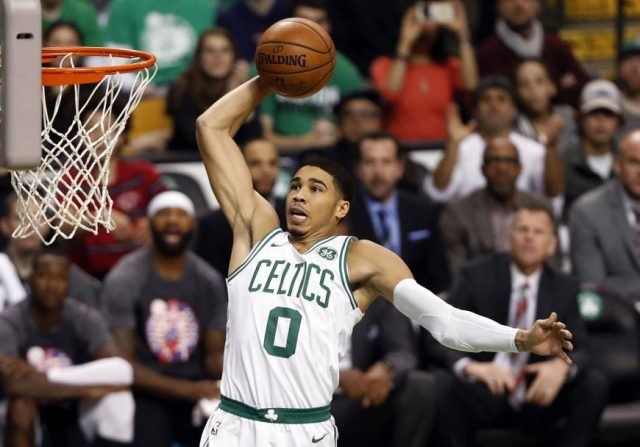# Celtics Win Streak Propelling Title Odds##### Celtics Win Streak Propelling Title Odds

After the Gordon Hayward injury and a couple of losses to begin the season, the Celtics’ title hopes took a hit. Though nobody besides the Warriors and Cavs really has a decent chance at winning the title, Boston’s implied probability fell from 7.69% to 4.58% within a week after the season began…not good.

Since then, they’ve been picking up the pieces. They’re not quite back to where they were before the season, but their 13 game win streak has proven that they are still legit without Hayward.

#### NBA Title

TeamNovember 15
(5Dimes)
October 17
(5Dimes)
July 7
(5Dimes)
July 1
(5Dimes)
June 28
(Westgate)
June 23
(5Dimes)
June 13
(BetOnline)
May 28
(Westgate)
Warriors-175-187-168-163-220-168-150-160
Cavs+540+515+350+350+300+330+450+250
Celtics+1390+1200+750+900+1500+1400+1600+1500
Rockets+1425+2150+1050+1050+1500+3300+2500+3000
Spurs+1625+1800+1000+1000+1200+1200+1000+1000
Thunder+2500+2150+3000+3000+8000+15000+5000+6000
Wizards+3750+9000+2800+2800+4000+5000+4000+3000
Timberwolves+6600+5000+3500+5000+6000+6600+10000+10000
Bucks+6600+8000+4500+4500+6000+6600+5000+6000
Raptors+6600+15000+5000+5000+8000+6600+5000+6000
Clippers+10000+19000+6600+6600+10000+6600+4000+3000
76ers+15000+19000+10000+10000+8000+25000+10000+10000
Heat+17500+24000+7000+8000+8000+12500+12500+10000
Nuggets+20000+20000+10000+10000+10000+25000+10000+10000
Grizzlies+20000+37000+10000+10000+20000+12500+8000+10000
Hornets+24500+50000+15000+15000+30000+40000+12500+30000
Jazz+25000+25000+3300+3300+10000+15000+5000+10000
Blazers+25000+31500+12500+12500+10000+15000+12500+10000
Pelicans+27500+22000+6000+6000+8000+10000+5000+8000
Pistons+30000+63000+15000+15000+20000+30000+12500+20000
Magic+30000+250000+50000+50000+100000+100000+100000+100000
Pacers+50000+125000+20000+20000+30000+20000+10000+10000
Lakers+100000+30000+10000+10000+10000+12500+10000+10000
Mavericks+100000+50000+15000+15000+20000+25000+12500+20000
Knicks+100000+100000+15000+15000+50000+30000+15000+30000
Nets+100000+250000+50000+50000+100000+100000+500000+100000
Kings+200000+125000+50000+50000+100000+100000+500000+100000
Hawks+200000+200000+20000+20000+20000+30000+15000+20000
Bulls+200000+250000+10000+10000+50000+20000+10000+10000
Suns+999999+125000+50000+50000+50000+100000+50000+50000

I wish this was like any other sport where you could see some legitimate odds changes, but this is the NBA, where one team has over a 60% chance of winning the title before the season even starts.

While other teams like the Rockets and Wizards have made big jumps on the odds list over the first month, their implied probabilities have only gone up by a couple percentage points.

The Cavs eeked out a win against the Knicks to claw back to the .500 mark, but despite their awful start to the season, their odds have hardly moved. Oddsmakers are likely assuming that once the playoffs come around, they’ll be the same Cavs team that has made it to the finals in three straight seasons. And unfortunately, they’ll probably be right.

Below are updated odds for each conference. At what point do the Cavs cease to be the odds-on favorite in the east?

#### Eastern Conference

Team5Dimes (11/15)5Dimes (10/17)BetOnline (7/6)Westgate (6/24)
Cleveland-140-165-140-250
Boston+310+245+275+450
Washington+1100+2200+1000+1200
Milwaukee+1725+2000+1400+1500
Toronto+1725+3100+1400+2000
Miami+4600+4000+4000+2000
Charlotte+6600+7500+5000+10000
Orlando+7500+25000+3300+40000
Detroit+7500+9000+5000+6000
Indiana+11000+14000+6600+10000
New York+20000+12000+5000+20000
Brooklyn+20000+25000+25000+40000
Atlanta+35000+21000+8000+6000
Chicago +35000+25000+10000+20000

#### Western Conference

Team5Dimes (11/15)5Dimes (10/17)BetOnline (7/6)Westgate (6/24)
Golden State-245-355-325-500
Houston+780+1100+600+1500
San Antonio+915+1000+600+650
Oklahoma City+1350+1100+2500+4000
Minnesota+3000+2600+2000+3000
Los Angeles Clippers+4750+8000+5000+2000
Denver+6600+8000+6600+5000
Memphis+6600+12500+6600+10000
Utah+7500+9000+4000+5000
Portland+7500+10500+8000+5000
New Orleans+8000+8300+4000+5000
Los Angeles Lakers+20000+9500+5000+5000
Dallas+20000+13500+10000+10000
Sacramento+35000+32500+350000+50000
Phoenix+100000+32500+350000+25000

Keep in touch with the news by following @SportsInsights and @ActionNetwork as we march onwards into a potentially interesting NBA season.

##### Mark Gallant

I'm the guy who does his job. You must be the other guy.